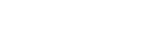# MATH211 - Survey of Calculus I

## General

MATH

211

00001829

Lecture

### Description

Ideas and concepts of calculus. Topics from differential and integral calculus of one variable. Applications to business, life sciences, economics, and other disciplines. Prereq.: MATH 106, MATH 111, MATH 112, MATH 115, MATH 196 or satisfactory math placement score.

### Notes

Go to www.stcloudstate.edu/mathplacement Placement req prior to Registration

3

3

No

Yes

04

No

## Learning Outcomes

### Outcome

Identify and make connections among functions represented in a variety of ways: graphical, numerical, analytical, or verbal.

### Outcome

Evaluate limits and solve problems applying the concept of limits.

### Outcome

Differentiate and integrate functions that are sum, difference, product, quotient, and composition of algebraic, exponential and logarithmic functions.

### Outcome

Set up and solve application problems involving the derivative (rate of change) and the integral (net change).

### Outcome

Find the extrema of functions using the First Derivative Test, Second Derivative Test, and Extreme Value Theorem.

### Outcome

Graph functions using the techniques of calculus.

### Outcome

Relate the derivative and the definite integral by way of the Fundamental Theorem of Calculus.

### Outcome

Communicate knowledge of functions, derivatives, and integrals, both orally (e.g. class discussions) and in writing (e.g. written assessments).

-

## Dependencies

No dependencies720 4th Avenue South
St. Cloud, MN 56301-4498
(320) 308-0121St. Cloud State University
A member of Minnesota State and committed to legal affirmative action, equal opportunity, access and diversity of its campus community (Full Statement).
© 2021 St. Cloud State University